Next: DEMASSIST Up: RELTIMING Previous: Output Description   Contents

# Implementation

The observation equations are given by the zero-degree polynomial model (y = A x):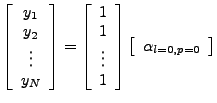(R1)

Where:
y contains the observed offsets in a certain direction.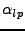denotes the unknown coefficients of the polynomial.

The least squares parameter solution is given by: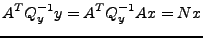(R2)

Where: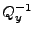is the (diagonal) covariance matrix of the observations.

The coefficients are estimated by factorization of the matrix N.

The inverse of matrix N is also computed to check the solution (stability) and to compute some statistics.

A check number is given (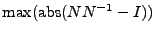) that gives a hint on the stability of the solution.

Leijen 2009-04-14